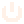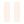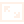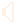# m4th.pro(gramming)

## make and play math video gamesResetPauseFullscreenSound

On keyboards, use the arrow keys, X (A-button), and Z (B-button).

# The goal

Use the arrow keys to transform the displayed matrix until you arrive at

$\begin{equation*}\pm \begin{pmatrix} 1 & 0 \\ 0 & 1 \end{pmatrix}.\end{equation*}$

If you make too many moves, you will lose a life! If you run out of time, you will also lose a life! You’ve got five lives before the game is over.

# How do I control this?

Moving “right” will add the second row to the first row, e.g.,

$\begin{equation*}\begin{pmatrix} 1 & -1 \\ 2 & \phantom{-}1 \end{pmatrix} \mapsto \begin{pmatrix} 3 & 0 \\ 2 & 1 \end{pmatrix}.\end{equation*}$

Moving “left” undoes this by subtracting the second row from the first row, e.g.,

$\begin{equation*}\begin{pmatrix} 3 & 0 \\ 2 & 1 \end{pmatrix} \mapsto \begin{pmatrix} 1 & -1 \\ 2 & \phantom{-}1 \end{pmatrix}.\end{equation*}$

Moving “up” and “down” are similar, but the roles of the first and second rows are exchanged. For instance, moving “up” will add the first row to the second row as in

$\begin{equation*}\begin{pmatrix} 1 & -1 \\ 2 & \phantom{-}1 \end{pmatrix} \mapsto \begin{pmatrix} 1 & -1 \\ 3 & \phantom{-}0 \end{pmatrix},\end{equation*}$

while moving “down” subtracts the first row from the second row as in

$\begin{equation*}\begin{pmatrix} 1 & -1 \\ 2 & \phantom{-}1 \end{pmatrix} \mapsto \begin{pmatrix} 1 & -1 \\ 1 & \phantom{-}2 \end{pmatrix},\end{equation*}$

One way to remember this is that right and left (the x-axis) affects the first row, while moving up and down (the y-axis) affects the second row. Right and up mean “add.”

# What’s with the background?

The background displays the upper half plane transformed by the displayed matrix regarded as a linear fractional transformation.